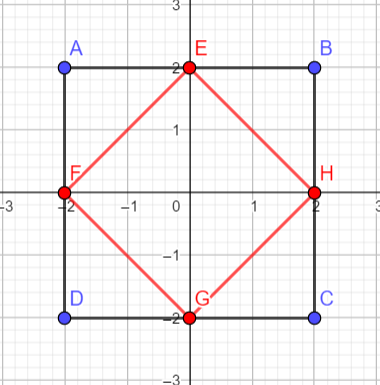# 各种距离

## 1. 欧几里得距离

$\begin{array}{c} \sqrt{(x_1-x_2)^2+(y_1-y_2)^2} \end{array}$

## 2. 曼哈顿距离

$\begin{array}{c} |x_1-x_2|+|y_1-y_2| \end{array}$

• ${|OB| \lt |OC|}$，则 ${|BC| \lt |OC|}$（曼哈顿距离），即连接 $O$$B$$C$ 三点的最短曼哈顿树为 ${O \rightarrow B \rightarrow C}$## 3. 切比雪夫距离

$\begin{array}{c} max(|x_1-x_2|, |y_1-y_2|) \end{array}$

###【曼哈顿距离与切比雪夫距离比较】## 4. 闵可夫斯基距离

$\begin{array}{c} \sqrt[p]{(x_1-x_2)^p+(y_1-y_2)^p} \end{array}$